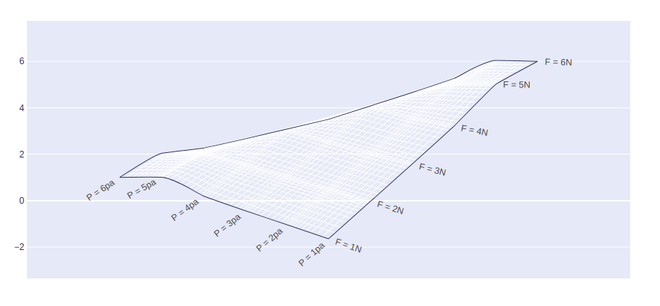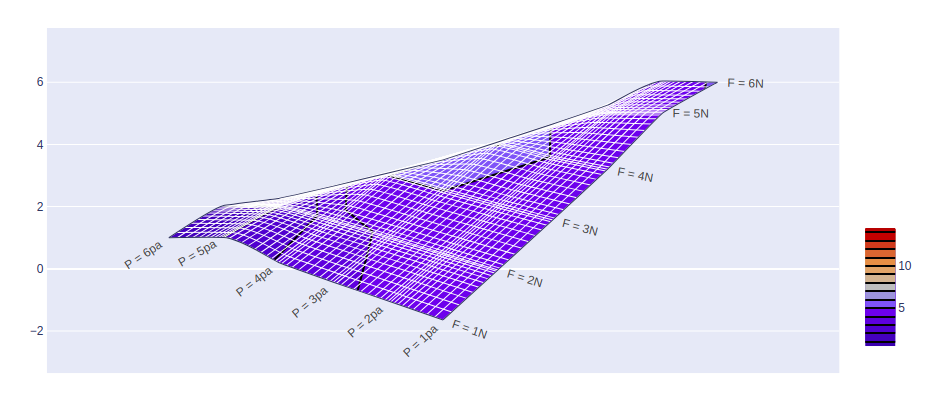Related Articles

# Carpet Contour Plot using Plotly in Python

• Last Updated : 01 Oct, 2020

A Plotly is a Python library that is used to design graphs, especially interactive graphs. It can plot various graphs and charts like histogram, barplot, boxplot, spreadplot, and many more. It is mainly used in data analysis as well as financial analysis. plotly is an interactive visualization library.

## Carpet Contour Plot

A carpet contour plot is a graphical technique for representing a 3-dimensional surface by plotting constant z slices, called contours, on a 2-dimensional format. X and Y attributes are used to set the x and y coordinates. In case the value of x is missing then it will form a cheater plot. A and B attributes should be used to save the values of the parameter.

Syntax: plotly.graph_objects.Contourcarpet( a=None,b=None,xaxis=None, yaxis=None, z=None)

Parameters:

Example:

## Python3

 `import` `plotly.graph_objects as go ` ` `  `fig ``=` `go.Figure(go.Carpet( ` `    ``a``=``[``1``, ``2``, ``3``, ``4``, ``5``, ``6``], ` `    ``b``=``[``6``, ``5``, ``4``, ``3``, ``2``, ``1``], ` `    ``y``=``[``1``, ``2``, ``3``, ``4``, ``5``, ``6``], ` ` `  `    ``aaxis``=``dict``( ` `        ``tickprefix``=``'F = '``, ` `        ``ticksuffix``=``'N'``, ` `        ``smoothing``=``0.2``, ` `        ``minorgridcount``=``10``, ` `    ``), ` `    ``baxis``=``dict``( ` `        ``tickprefix``=``'P = '``, ` `        ``ticksuffix``=``'pa'``, ` `        ``smoothing``=``0.4``, ` `        ``minorgridcount``=``9``, ` `    ``) ` `)) ` ` `  `fig.show() `

Output:In plotly, contours can be added by using the go.Contourcarpet() method of graph_object class. Contours should be added in such a way that the graph body should be in the proper segment.

Example:

## Python3

 `import` `plotly.graph_objects as go ` ` `  `fig ``=` `go.Figure() ` ` `  `fig.add_trace(go.Contourcarpet( ` `    ``a ``=` `[``1``, ``2``, ``3``, ``4``, ``5``, ``6``], ` `    ``b ``=` `[``6``, ``5``, ``4``, ``3``, ``2``, ``1``], ` `    ``z ``=` `[``1``, ``1.96``, ``5``, ``6.1028``, ``4``, ``5.0625``], ` `    ``autocontour ``=` `False``, ` `    ``contours ``=` `dict``( ` `        ``start ``=` `1``, ` `        ``end ``=` `14``, ` `        ``size ``=` `1` `    ``), ` `    ``line ``=` `dict``( ` `        ``width ``=` `2``, ` `        ``smoothing ``=` `0` `    ``), ` `    ``colorbar ``=` `dict``( ` `       ``len` `=` `0.4``, ` `        ``y ``=` `0.25` `    ``) ` `)) ` ` `  ` `  `fig.add_trace(go.Carpet( ` `    ``a``=``[``1``, ``2``, ``3``, ``4``, ``5``, ``6``], ` `    ``b``=``[``6``, ``5``, ``4``, ``3``, ``2``, ``1``], ` `    ``y``=``[``1``, ``2``, ``3``, ``4``, ``5``, ``6``], ` ` `  `    ``aaxis``=``dict``( ` `        ``tickprefix``=``'F = '``, ` `        ``ticksuffix``=``'N'``, ` `        ``smoothing``=``0.2``, ` `        ``minorgridcount``=``10``, ` `    ``), ` `    ``baxis``=``dict``( ` `        ``tickprefix``=``'P = '``, ` `        ``ticksuffix``=``'pa'``, ` `        ``smoothing``=``0.4``, ` `        ``minorgridcount``=``9``, ` `    ``) ` `)) ` ` `  `fig.show() `

Output:Attention geek! Strengthen your foundations with the Python Programming Foundation Course and learn the basics.

To begin with, your interview preparations Enhance your Data Structures concepts with the Python DS Course. And to begin with your Machine Learning Journey, join the Machine Learning – Basic Level Course

My Personal Notes arrow_drop_up
Recommended Articles
Page :